• 下表列出了中国某年按行业分的全部制造业国有企业及规模以上制造业国有企业的工业总产值Y，资产合计K及职工人数L。 (l）利用上述资料，进行回归分析。 (2）回答：中国该年的制造业总体呈现规模报酬不变状态吗？ ...
下表列出了中国某年按行业分的全部制造业国有企业及规模以上制造业非国有企业的工业总产值Y，资产合计K及职工人数L。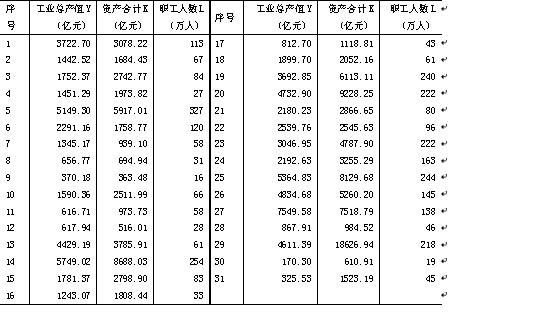(l）利用上述资料，进行回归分析。
(2）回答：中国该年的制造业总体呈现规模报酬不变状态吗？
格式：第一次上机实验+姓名+学号。
发送内容：包括结论和stata工作文件(其它软件格式也行)。
时间：本周周六上课之前。
*****作答
将模型设定为，对数变换为
1）进行回归分析
python代码：
1.import numpy as np
2.import pandas as pd
3.import statsmodels.api as sm
4.#导入数据
5.df = pd.read_csv("上机实验一.csv")
6.df=np.log(df)
7.#多元线性回归
8.model = sm.OLS(df['工业总产值Y（亿元）'], sm.add_constant(df.iloc[:, 1:])).fit()
9.Print(model.summary())

结果：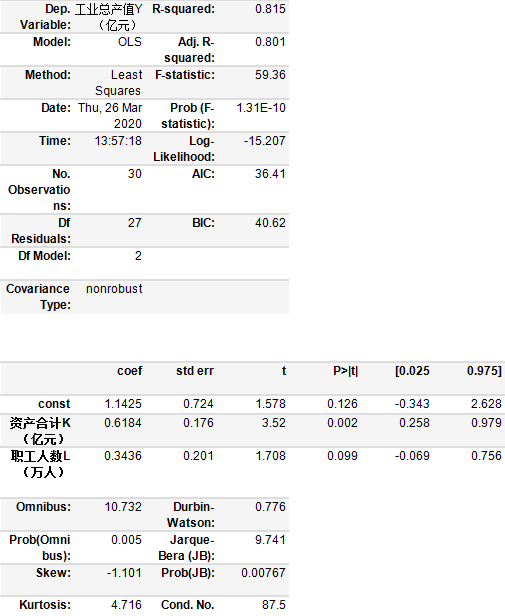于是，样本回归方程为：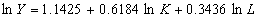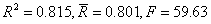从回归结果可以看出，模型的拟合度较好，在显著性水平 0.1 的条件下，各项系数均通过了 t 检验。从 F 检验可以看出，方程对 Y 的解释程度较少。
表明，工业总产值对数值的 80.1%的变化可以由资产合计对数与职工的对数值的变化来解释，但仍有 20.4%的变化是由其他因素的变化影响的。
2） 从上述回归结果看，，即资产与劳动的产出弹性之和近似为 1，表明中国制造业在该年基本呈现规模报酬不变的状态。
3） 回归诊断图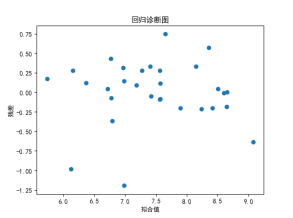若因变量与自变量线性相关，残差值应该和拟合值没有任何的系统关联，呈现出围绕着0随机分布的状态。从图来看，这一假定基本上满足。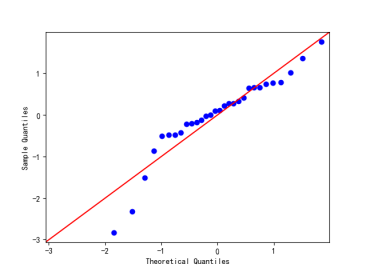当因变量成正态分布时，那么模型的残差项应该是一个均值为0的正态分布。正态Q_Q图(Normal Q-Q)是在正态分布对应的值下，标准化残差的概率图，若满足正态性假设，那么图上的点应该落在一条直线上，基本满足。


展开全文• 本课程主要讲述如何使用python进行线性回归与非线性回归分析，包括： 基于statsmodel的线性回归方法 基于sklearn的线性回归方法 基于Numpy的一元多项式非线性回归方法 基于sklearn的多元多项式非线性回归方法 基于...大数据就业前景 工业大数据 云计算 云计算是什么
• 概念： 一元非线性回归分析（Univariate Nonlinar Regression）:在回归分析中，只包含一个自变量和一个因变量，...求解：将一元非线性回归方程转为多元线性回归方程，例如： 下面是代码演示： 首先导入数据集，取出...
概念：
一元非线性回归分析（Univariate Nonlinar Regression）:在回归分析中，只包含一个自变量和一个因变量，且二者的关系可以用一条曲线表示，则成为一元非线性分析。
案例：游戏等级和木材消耗的关系。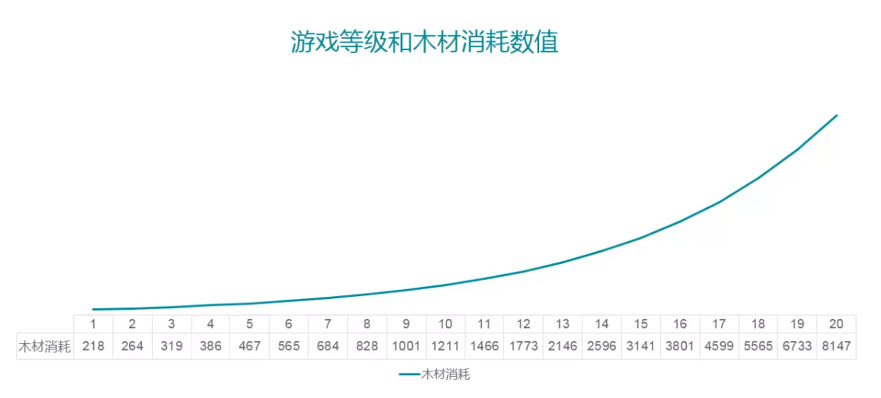木材消耗和游戏等级呈一条曲线关系，探究木材消耗和游戏等级之间的非线性关系。
求解：将一元非线性回归方程转为多元线性回归方程，例如：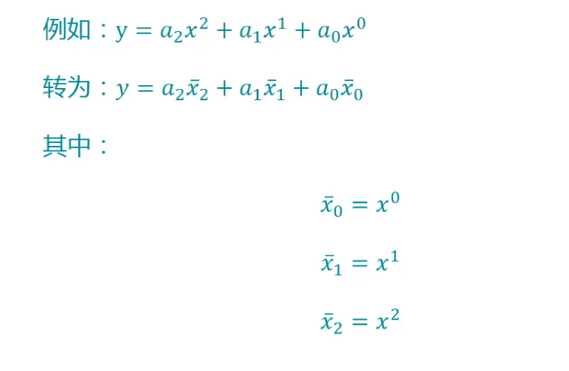下面是代码演示：
首先导入数据集，取出自变量和因变量。
import pandas
import matplotlib;

data = pandas.read_csv(
r'C:\Users\www12\Desktop\DA\pythonDM\4.3\data.csv'
)
#取出自变量和因变量
x = data[["等级"]]
y = data[["资源"]]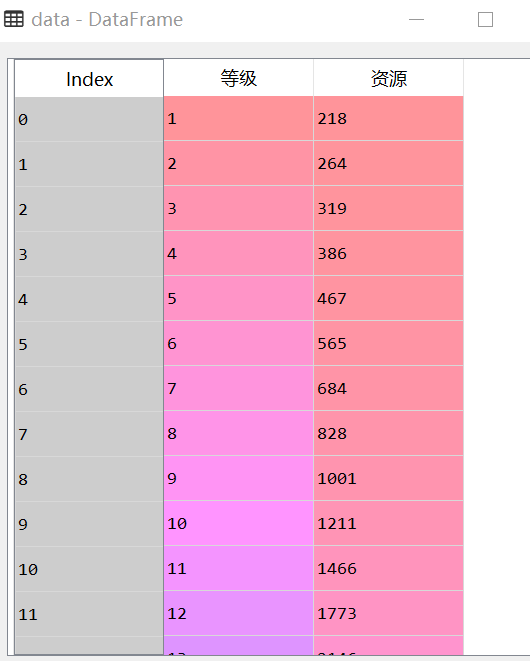然后，绘制出两个变量之间的散点图：
font = {
'family' : 'SimHei'
};
matplotlib.rc('font', **font);
matplotlib.rcParams['axes.unicode_minus'] = False

from pandas.tools.plotting import scatter_matrix;

scatter_matrix(
data[["等级", "资源"]],
alpha=0.8, figsize=(10, 10), diagonal='kde'
)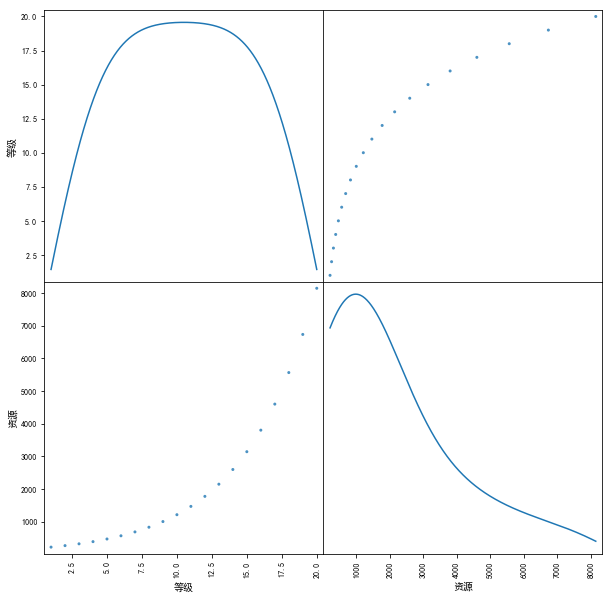可以看出是一元二次方程的曲线，我们画一个一元二次方程曲线来比较一下：
import numpy;
x_ = numpy.arange(-10, 10, 0.01);
y_ = x_**2

from matplotlib import pyplot as plt;

plt.figure();
plt.title('等级与资源')
plt.xlabel('等级')
plt.ylabel('资源')
plt.grid(True)
plt.plot(x_, y_, 'k.')
plt.show()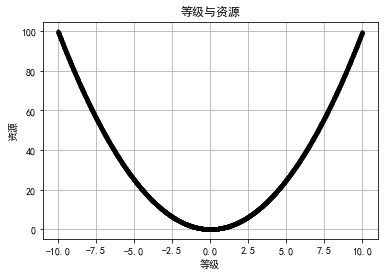然后我们要将一元非线性回归方程转化为多元线性回归方程：
用到方法是PolynomialFeatures（）方法
导入包
from sklearn.linear_model import LinearRegression
from sklearn.preprocessing import PolynomialFeatures
x

pf = PolynomialFeatures(degree=2)  #   传入degree参数，表示一元二次方程
x_2_fit = pf.fit_transform(x)

x_2_fit
#查看x_2_fit：
array([[  1.,   1.,   1.],
[  1.,   2.,   4.],
[  1.,   3.,   9.],
[  1.,   4.,  16.],
[  1.,   5.,  25.],
[  1.,   6.,  36.],
[  1.,   7.,  49.],
[  1.,   8.,  64.],
[  1.,   9.,  81.],
[  1.,  10., 100.],
[  1.,  11., 121.],
[  1.,  12., 144.],
[  1.,  13., 169.],
[  1.,  14., 196.],
[  1.,  15., 225.],
[  1.,  16., 256.],
[  1.,  17., 289.],
[  1.,  18., 324.],
[  1.,  19., 361.],
[  1.,  20., 400.]])

#然后就转换为二元线性回归方程了
lrModel = LinearRegression()
lrModel.fit(x_2_fit, y)  #训练模型

lrModel.score(x_2_fit, y)  #模型评分

x_2_predict = pf.fit_transform([, , ])   #预测的时候自变量需要经过转换再预测
lrModel.predict(x_2_predict)   #模型预测

预测结果：
array([[ 8449.29298246],
[ 9490.99924812],
[10594.83697881]])

API总结：
一元n次方程，转多元线性回归方程：
pf = PolynomialFeatures(degree=2)
参数说明：
degree ：回归方程的次数
转换方法：
x_2_fit = pf.fit_transform(x)


展开全文• ## python实现线性回归

千次阅读 2018-07-21 19:09:30
线性回归模型是最简单的机器学习模型，基础可以从线性回归模型开始入手，慢慢地过渡到非线性回归以及神经网络模型。 1.概念  在统计学中，线性回归是利用称为线性回归方程的最小平方函数对一个或多个自变量和因...
       线性回归模型是最简单的机器学习模型，基础可以从线性回归模型开始入手，慢慢地过渡到非线性回归以及神经网络模型。

1.概念

在统计学中，线性回归是利用称为线性回归方程的最小平方函数对一个或多个自变量和因变量进行建模的一种回归分析。这种函数是一个或多个称为回归系数的模型参数的线性组合。只有一个自变量的情况称为简单回归，大于一个自变量情况叫做多元回归。    来源：百度百科

2.线性回归

简单回归：一个自变量输入，Y~X是一对一的关系，映射到几何上来说就是二维坐标系的直线方程，可表示为Y=X，通过训练得到的过程。

多元回归：多个自变量，改变维度的大小。即： ,    ， 表示偏移量，表示空间分布。因为进行python运算时要循环，当X的维度增多时，循环的工作量就增大，对内存和cpu消耗也大。实际上，Y和X是一种矩阵形式乘，即  ,    ,   ，同样从训练中的得到参数  的矩阵向量的过程

3.最小二乘法 【通过向量运算】

大多情况下都是求近似最优解，即满足估计值于实际值的方差最小时的参数解，可以表示为：

函数模型：

损失函数：   ， 简单形式为： , 公式是用欧几里得距离求解空间向量距离

要使损失函数最小，就要对函数求导计算，通过一系列数学推导，可以得到一个标准方程：

参数计算：

4.梯度下降算法

直接向量运算存在的问题：（1）矩阵必须是满秩的，如果不是，python也能模糊处理（2）运算性能，矩阵扩大化后，量级增大对性能影响明显。

梯度下降不仅限于线性回归，非线性和神经网络同样适用。

在线性回归中，  通过  学习速率在每个J( ) 减小的维度上进行一个 不定式  参数的递减，不断更新  的值直到 J( ) 收敛，达到最小值。类比于下山，每次尝试找比这个位置低的位置，一步一步到达山底（即我们求的最小值）

5.应用

在数学方面进行多元一次方程的求解，即线性方程的求解；

在金融中对简单金融模型的预测。如资本资产定价模型利用线性回归以及Beta系数的概念分析和计算投资的系统风险。

在医学方面对流行病的预测。如有关吸烟对死亡率和发病率影响的早期证据来自采用了回归分析的观察性研究。

等等，线性回归模型有助于帮助了解工作环境中的关系，简单得对一些变量进行观察分析从而有所收获

6.demo

import numpy as np
from numpy.linalg import inv   #求矩阵的逆
from numpy import dot  #矩阵点乘
from numpy import mat  #矩阵
import pandas as pd
from pandas import read_csv

# pwd = os.getcwd()
# os.chdir(os.path.dirname(path))
# dataset = pd.read_csv(os.path.basename(path),encoding='gbk')
# os.chdir(pwd)
dataset = pd.read_csv('data.csv')
# print(dataset)

temp=dataset.iloc[:,2:5]
temp['x0']=1
X=temp.iloc[:[3,0,1,2]]
# print(X)
Y=dataset.iloc[:,1].values.reshape(len(Y),1)   //len(Y)=150
# print(Y)
theta = dot(dot(inv(dot(X.T,X)),X.T),Y)  #最小二乘法
print(theta)

theta=np.array([1.,1.,1.,1.,1.]).reshape(4,1)
alpha=0.1
temp=theta
x0=X.iloc[:,0].values.reshape(len(Y),1)
x1=X.iloc[:,1].values.reshape(len(Y),1)
x2=X.iloc[:,2].values.reshape(len(Y),1)
x3=X.iloc[:,3].values.reshape(len(Y),1)
for i in rang(10000):
temp=theta-alpha*np.mean(dot(X,theta)-Y)*x0
temp=theta-alpha*np.mean(dot(X,theta)-Y)*x1
temp=theta-alpha*np.mean(dot(X,theta)-Y)*x2
temp=theta-alpha*np.mean(dot(X,theta)-Y)*x3
theta=temp
print(theta)

这个代码是照着视频敲的，一开始提示不存在data.csv时以为是我没安装pandas库，当我用命令行安装完后还是提示不存在，然后上百度搜，换了注释的写法，依旧不对，再对path进行修改，我才发现我python里没有data.csv ，百度也没找到正确解答的，如果盆友们能用这个代码的话，帮忙反馈一下我出现的问题在哪里，万分感谢！ /鞠躬

记录来源：观看慕课网《Python实现线性回归》视频，同时参照https://www.cnblogs.com/wallacup/p/6017378.html ，以及百度百科，如有问题，还望多多指教
展开全文最小二乘法 梯度下降算法
• matlab多元参数非线性回归模型代码NEU 337-2020年Spring（53760） 现代神经科学的程序设计和数据分析 !!! 请在第一类之前安装Python和JupyterLab （请参阅下面的说明）。 如果遇到任何麻烦，请不要踩踏。 首先，在...
• 在一元线性回归中，输入特征只有一维， hθ=θ0+x1θ1h_{θ}=θ_0 + x_1θ_1, 对于多元特征，假设...对于非线性的一维数据，用线性回归拟合结果并不好，可以采用多项式回归，手动增加特征，例如如下4种多项式拟合 hθ
在一元线性回归中，输入特征只有一维， hθ=θ0+x1θ1<!--//--><![CDATA[//><!--
h_{θ}=θ_0 + x_1θ_1
//--><!]]>, 对于多元特征，假设函数推广到了 hθ=θ0+x1θ1+x2θ2+x3θ3+...+xmθm<!--//--><![CDATA[//><!--
h_{θ}=θ_0 + x_1θ_1+ x_2θ_2+ x_3θ_3+...+ x_mθ_m
//--><!]]>
对于非线性的一维数据，用线性回归拟合结果并不好，可以采用多项式回归，手动增加特征，例如如下4种多项式拟合 hθ=θ0+θ1x1+θ2x21<!--//--><![CDATA[//><!--
h_{θ}=θ_0 + θ_1x_1+θ_2x_1^2
//--><!]]>      (1) hθ=θ0+θ1x1+θ2x21θ2+θ3x31<!--//--><![CDATA[//><!--
h_{θ}=θ_0 + θ_1x_1+θ_2x_1^2θ_2+θ_3x_1^3
//--><!]]>     (2) hθ=θ0+θ1x1+θ2log(x1)<!--//--><![CDATA[//><!--
h_{θ}=θ_0 + θ_1x_1+θ_2log(x_1)
//--><!]]>                    (3) hθ=θ0+θ1x1+θ2x√1<!--//--><![CDATA[//><!--
h_{θ}=θ_0 + θ_1x_1+θ_2\sqrt x_1
//--><!]]>        (4)
我们可以将多项式回归转换为多元回归，例如在式(1)中，可以令x21<!--//--><![CDATA[//><!--
x_1^2
//--><!]]>为x2<!--//--><![CDATA[//><!--
x_2
//--><!]]>，此时假设函数为 hθ=θ0+θ1x1+θ2x2<!--//--><![CDATA[//><!--
h_{θ}=θ_0 + θ_1x_1+θ_2x_2
//--><!]]> ，
根据梯度下降求出θ0，θ1，θ2<!--//--><![CDATA[//><!--
θ_0，θ_1，θ_2
//--><!]]> 。
在上一篇博文一元线性回归中，
(http://blog.csdn.net/murphy_coolcoder/article/details/78946003)
最终拟合出的曲线如图所示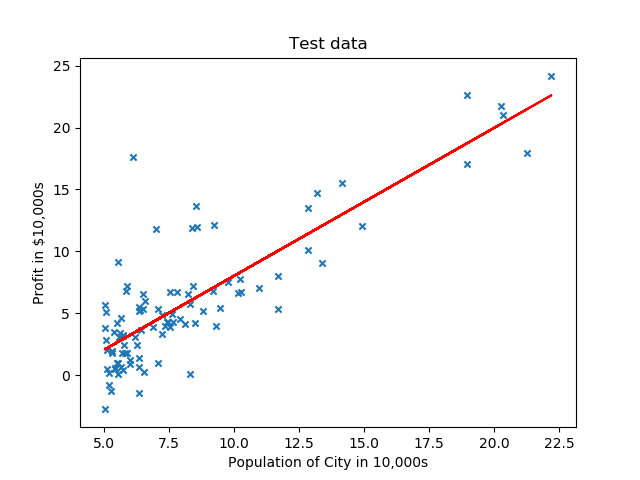如果我们想采用如下曲线拟合 hθ=θ0+θ1x1+θ2log(x1)<!--//--><![CDATA[//><!--
h_{θ}=θ_0 + θ_1x_1+θ_2log(x_1)
//--><!]]>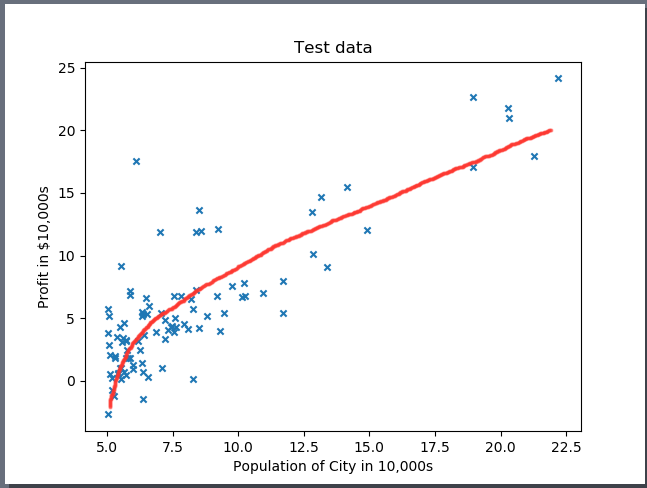或者如下二次函数拟合 hθ=θ0+θ1x1+θ2x21<!--//--><![CDATA[//><!--
h_{θ}=θ_0 + θ_1x_1+θ_2x_1^2
//--><!]]>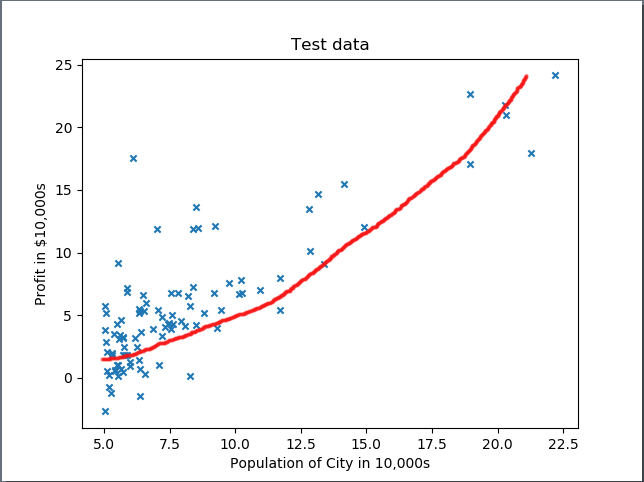则需要对输入数据增加一个对应的特征X2<!--//--><![CDATA[//><!--
X_2
//--><!]]>, 若采用 log则 X2=log(X1)<!--//--><![CDATA[//><!--
X_2 = log(X_1)
//--><!]]>,若采用二次函数则 X2=X21<!--//--><![CDATA[//><!--
X_2 = X_1^2
//--><!]]>,然后进行梯度下降，得到 θ0，θ1，θ2<!--//--><![CDATA[//><!--
θ_0，θ_1，θ_2
//--><!]]> 。
这里有几个trick要注意，
1).增加了一个特征之后，我们实际上将数据上升到3维空间，在做梯度下降之前，我们对训练样本先要进行归一化，尤其如果是高次多项式，特征之间数值差别很大，不做归一化梯度下降会很慢，而且还容易停止在局部最优而不是全局最优，不利于后续数值处理。
2).由于多项式回归是单一特征的多项式和，因此可以在二维空间展示出来，就如上图二所示。要在二维空间展示多项式曲线，对于输入数据要先做归一化，再增加特征向量。但此时会有一个问题存在，如果是多项式是log函数，在使用z-score归一化的情况下，没法在二维空间展示。因为采用z-score归一化的一维特征Norm_X_1<!--//--><![CDATA[//><!--
Norm_X_1
//--><!]]>会有负值存在，此时在此基础之上增加log(Norm_X_1)<!--//--><![CDATA[//><!--
log(Norm_X_1)
//--><!]]>特征会无效. 只能在三维空间展示。当然如果可以采用某种归一化方法，使其一维特征经过归一化后没有负值和0值存在，那么二维特征log(Norm_X_1)<!--//--><![CDATA[//><!--
log(Norm_X_1)
//--><!]]>便可以运算，则也可以在二维空间展示出来，本文不做深入探讨。
3). 对于测试样本，测试样本的归一化参数要使用训练样本的归一化参数。如训练样本采用z-score归一化方法， 均值u1, 方差sigma1。 对于测试样本，测试样本的归一化参数不应使用自身的均值u2和方差sigma2而应使用训练样本的均值u1 和方差sigma1 作为归一化参数。
4). 在使用Matplotlib 绘制拟合函数时，建议使用散点图绘制，可以避免参数顺序变化带来的图像错乱。

源代码如下，数据集
训练样本数据https://pan.baidu.com/s/1nuM66mt
note： 本代码对数据集采用8：2的比例作为训练样本与测试样本，由于使用了np.random.shuffle()函数，每次运行的结果不尽相同，如要想要修改训练样本与测试样本的比例或者固定样本选择顺序，可以修改类中 sampleDivde 方法，参数“ratio”可以调整比例，注释掉np.random.shuffle()函数可以固定训练样本选择顺序。

import numpy as np
import matplotlib.pyplot as plt
from mpl_toolkits.mplot3d import Axes3D
from scipy.interpolate import spline

class LinearRegression:
def sampleDivde(self,data_X,data_Y,feature_num,ratio):
combineMatix = np.append(data_X.T,data_Y).reshape(feature_num+1,data_X.shape).T
np.random.shuffle(combineMatix)
m = int(data_X.shape*ratio)
train_X = combineMatix[:m,:feature_num]
train_Y = combineMatix[:m,feature_num]
test_X =combineMatix[m:,:feature_num]
test_Y = combineMatix[m:,feature_num]
return train_X,train_Y,test_X,test_Y
def featureScale(self, data_X):
u = np.mean(data_X,0)
sigma = np.std(data_X,0)
newData = (data_X - u)/sigma
return newData, u, sigma
def testScale(self,data_X,u,sigma):
newData = (data_X - u) / sigma
return newData

def biasAdd(self, data_X, feature_num):
biasX = np.ones(data_X.shape)
newX = np.append(biasX, data_X.T).reshape(feature_num + 1, data_X.shape).T
return newX
def featureAdd(self, data_X, model, feature_num):
if model == 0:
return data_X
if model == 1:
feature_add = np.log2(data_X)
elif model == 2:
feature_add = np.sqrt(data_X)
elif model == 3:
feature_add = np.power(data_X,2)
X = np.append(data_X, feature_add).reshape(feature_num + 1, data_X.shape).T
return X
def costFunc(self,theta,data_X,data_Y):
m = data_X.shape
J = np.dot((np.dot(theta,data_X.T).T- data_Y).T,np.dot(theta,data_X.T).T-data_Y)/(2*m)
return J
def gradientDescent(self,theta,data_X,data_Y,alpha,num_iters):
m = data_X.shape
for i in range(num_iters):
theta = theta - np.dot(data_X.T,np.dot(theta.T,data_X.T).T- data_Y)*alpha/m
return theta
def error(self,theta,test_X,Y_label):
test_Y = np.dot(theta,test_X.T)
error = sum(test_Y-Y_label)/Y_label.shape
return error
def learningRateCheck(self,theta,data_X,data_Y,alpha):
m = data_Y.shape
j_X = []
j_Y = []
for i in range(0,100,5):
j_X.append(i)
for j in range(i):
theta = theta - np.dot(data_X.T, np.dot(theta.T, data_X.T).T - data_Y) * alpha / m
j_Y.append(self.costFunc(theta,data_X,data_Y))
xNew = np.linspace(min(j_X), max(j_X), 300)
ySmooth = spline(j_X,j_Y,xNew)
plt.plot(xNew,ySmooth, color = 'red')
plt.show()
if __name__ =='__main__':
path = 'Housedata.txt'
fr = open(path, 'r+')
x = []
y = []
for line in fr:
items = line.strip().split(',')
x.append(float(items))
y.append(float(items))
test = LinearRegression()
data_X = np.array(x)
data_Y = np.array(y)
a = data_X
z = data_Y
train_X, train_Y, test_X, test_Y = test.sampleDivde(data_X, data_Y,1,0.8) #no bias
#Linear regression data pre-processing
norm_train_X,u,sigma = test.featureScale(train_X)
biasnorm_train_X = test.biasAdd(norm_train_X,1)
norm_test_X = test.testScale(test_X,u,sigma)
biastest_X = test.biasAdd(norm_test_X,1)
# gradient Descent
theta = np.zeros(biasnorm_train_X.shape)
theta_Results = test.gradientDescent(theta, biasnorm_train_X, train_Y, 0.1, 1500)
#Plot data
X = train_X
Y = np.dot(theta_Results,  biasnorm_train_X.T)
plt.subplot(211)
plt.plot(X, Y, color='red')
plt.scatter(data_X, data_Y, s=20, marker="x") #plot raw data
plt.title('Linear fit')

#Polyomial Regression#
#************1. Quadratic fit*********
# train data used for train
Q_feature_add_train_X = test.featureAdd(train_X, 3, 1)  # 3 in featureAdd function represent squre
Q_norm_train_X, Q_u, Q_sigma = test.featureScale(Q_feature_add_train_X)
Q_biasnorm_train_X = test.biasAdd(Q_norm_train_X, 2)
# test data used for train
Q_feature_add_test = test.featureAdd(test_X, 3, 1)
Q_norm_test = test.testScale(Q_feature_add_test, Q_u, Q_sigma)
Q_bias_test_X = test.biasAdd(Q_norm_test, 2)
#gradient Descent
theta = np.zeros(Q_biasnorm_train_X.shape)
Q_theta_Results = test.gradientDescent(theta, Q_biasnorm_train_X, train_Y, 0.1, 1500)
#data used for plot,which is featureScale first, than add feature X2.
Q_norm_X,p_u,p_sigma = test.featureScale(train_X)
Q_feature_add_norm_X = test.featureAdd(Q_norm_X, 3, 1)
Q_biasnorm_X = test.biasAdd(Q_feature_add_norm_X, 2)
# Plot data
X = train_X
Y = np.dot(Q_theta_Results, Q_biasnorm_X.T)
plt.subplot(212)
plt.scatter(X, Y, color='red')
plt.scatter(data_X, data_Y, s=20, marker="x")  # plot raw data
plt.title('Quadratic fit')
plt.show()

# ************3. Log fit*********
# train data used for train
L_feature_add_train_X = test.featureAdd(train_X, 1, 1)  # 1 in featureAdd function represent  log
L_norm_Train_X,L_u,L_sigma = test.featureScale(L_feature_add_train_X)
L_biasTrain_X = test.biasAdd(L_norm_Train_X, 2)
# test data used for train
L_feature_add_test_X = test.featureAdd(test_X, 1, 1)
L_norm_Test_X = test.testScale(L_feature_add_test_X,L_u,L_sigma)
L_biasTest_X = test.biasAdd(L_norm_Test_X, 2)
# gradient Descent
theta = np.zeros(L_biasTrain_X.shape)
L_theta_Results = test.gradientDescent(theta, L_biasTrain_X, train_Y, 0.2, 1500)
# Plot 3D data
ax = plt.subplot(111, projection='3d')
X = train_X
Y = np.log2(train_X)
Z = np.dot(L_theta_Results, L_biasTrain_X.T)
ax.scatter(X,Y,Z, color='g')
ax.scatter(X,Y,train_Y, c='r')  # 绘制数据点
ax.set_zlabel('Z')  # 坐标轴
ax.set_ylabel('Y')
ax.set_xlabel('X')
plt.show()

######Error  check########
error = test.error(theta_Results, biastest_X, test_Y)
print("Linear regression error is:",error)
Q_error = test.error(Q_theta_Results, Q_bias_test_X, test_Y)
print("Quadratic Polyomial Regression error is:",Q_error)
L_error = test.error(L_theta_Results, L_biasTest_X, test_Y)
print("Log Polyomial Regression error is:",L_error)

二次拟合结果与一元线性回归拟合比较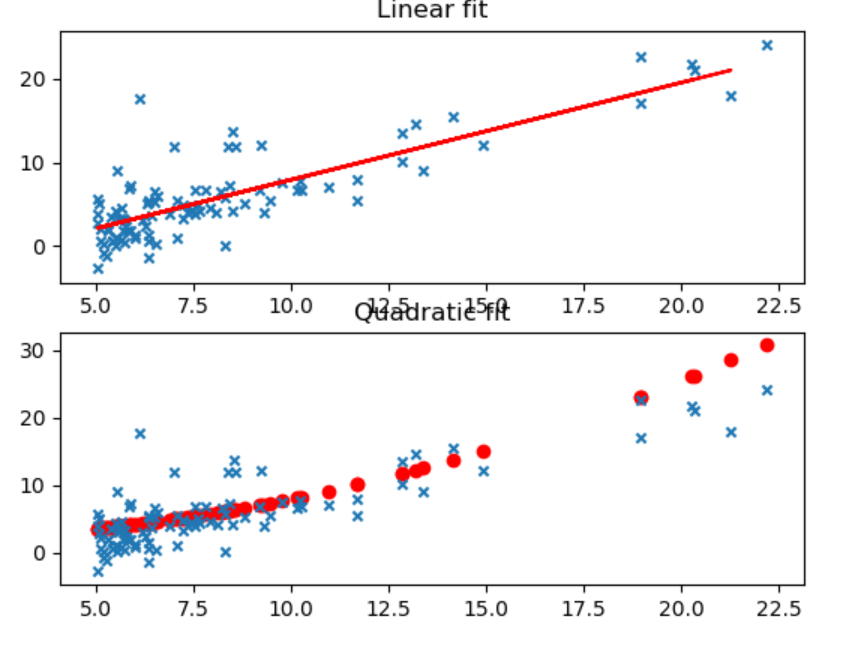Log 拟合三维图像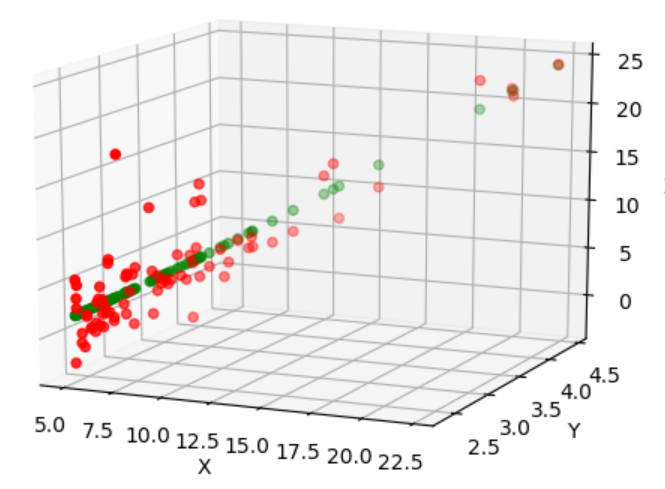拖动三维图像，可以看出X与Y 的对数关系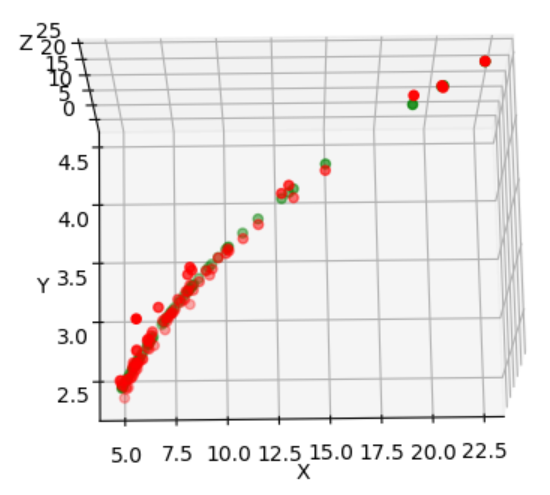由于采取了随机策略，由于训练样本选择的不同，三种方法的误差比较也不尽相同。某些情况下Log拟合最优，某些情况下线性回归反而表现要好。这是与数据集分不开的。就本实例的数据集来说，三种拟合方式差别不大。对应于不同的数据集，采用不同的方式，甚至更高次的多项式拟合，要根据具体问题来分析。本文只是给简要分析了一下多项式拟合和多元线性回归二者之间的关系以及我在调试代码过程中所遇到的问题，以供参考。
展开全文• matlab多元参数非线性回归模型代码Coursera机器学习与实践 记录了的研究，但添加了一些加强学习的实践。 目录 第1周 介绍 Machine Learning definition ：如果某计算机程序在T上的性能（由P衡量）随着经验E的提高而...
• 要理解线性回归，我们的对线性和回归的基本概念进行回顾。 线性：两个变量之间存在一次方函数关系即...按照自变量和因变量之间的关系类型，可分为线性回归分析和非线性回归分析。 一、线性回归算法的基本流程...
• matlab多元参数非线性回归模型代码完善的机器学习：笔记，练习和Jupyter笔记本 在下面，您将找到补充第二版《机器学习精炼》（由剑桥大学出版社出版）的一系列资源。 目录 小部件样本和我们的教学法 我们相信，只有...
• 回归分析 回归分析是确定两种或两种以上变量间相互依赖的定量关系的一种统计分析方法。 按照涉及的变量的多少，分为...1.一元、多元以及非线性回归 一元线性回归方程： 多元线性回归方程： 非线性回归方...
• matlab多元参数非线性回归模型代码动态主体间同步以响应悲伤音乐的脚本和分析步骤 用于分析和创建图像的所有脚本，这些数据在Sachs，ME，Habibi，A.，Damasio，A.，＆Kaplan，JT（2019）中提供。 动态主体间神经同步...
• Python3入门机器学习 3.1 简单线性回归 1.线性回归算法的优点： 解决回归问题 ...样本特征有多个称为多元线性回归。 3.一类机器学习算法的基本思路： 以上的过程就是一类机器学习算法的基本思算法 机器学习 人工智能
• 本次推文介绍用线性模型处理回归问题。... 紧接着，介绍多项式回归分析（polynomial regression 问题），一种具有非线性关系的多元线性回归问题。 最后，介绍如果训练模型获取目标函数最小化的参数值。 在研究一个大数机器学习 sklearn
• Python数据分析——第10周 回归分析 回归分析：通过建立模型来研究变量之间相互...多元非线性回归： 含有定性变量的回归：少年组、青年组、老年组，分别是0,、1、2 函数关系与相关关系  函数关系：确定性关系，y=...
• 梯度下降算法是迭代法的一种,可以用于求解最小二乘问题(线性和非线性都可以)，在求解机器学习算法的模型参数，即无约束优化问题时，梯度下降（Gradient Descent）是最常采用的方法之一。 在机器学习中，基于基本的...人工智能 机器学习 梯度下降算法
• 多元回归分析(Multiple Regression Analysis)是指在相关变量中将一个变量视为因变量，其他一个或多个变量视为自变量，建立多个变量之间线性或非线性数学模型数量关系式并利用样本数据进行分析的统计分析方法。...
• sklearn的波士顿房价数据是经典的回归数据集。在MOOC的课程《用Python玩转数据》...同时使用非线性回归模型来拟合（暂时还没想好用哪个？xgboost，还是SVM？）。 2、使用tensorflow建立回归模型拟合。 一、使用...
• 可分为线性回归分析和非线性回归分析。如果在回归分析中，只包括一个自变量和一个因变量，且二者的关系可用一条直线近似表示，这种回归分析称为一元线性回归分析。如果回归分析中包括两个或两个以上的自变量，且因...
• 何为回归分析： 回归分析（regression ...按照自变量和因变量之间的关系类型，可分为线性回归分析和非线性回归分析。 在大数据分析中，回归分析是一种预测性的建模技术，它研究的是因变量（目标）和自变量（预...
•     非线性回归一般可以分为一元非线性回归和多元非线性回归。     一元非线性回归是指两个变量-----一个自变量，一个因变量之间呈现非线性关系，如双曲线、二次曲线、三(多)次曲线、幂曲线、指数曲线、对数...机器学习
• 其实质上是把预报问题按状态空间的取值进行分类，用分段的线性回归模式来描述总体非线性预报问题。 多元门限回归的建模步骤就是确实门限变量、率定门限数L、门限值及回归系数的过程，为了计算方便，这里采用二分割...
• ## python实现门限回归

千次阅读 2018-10-28 15:16:13
门限回归模型(Threshold Regressive Model，简称TR模型或TRM)的基本...其实质上是把预报问题按状态空间的取值进行分类，用分段的线性回归模式来描述总体非线性预报问题。多元门限回归的建模步骤就是确实门限变量、...
• 　一元线性回归、多元线性回归、Logistic回归、广义线性回归、非线性回归的关系 　什么是极大似然估计 　逻辑斯谛回归(Logistic回归) 　多类分类Logistic回归 　Python代码(sklearn库) 一元线性...
• 多项式回归是一种线性回归形式... 通常的多元线性回归分析的假设是所有自变量都是独立的。在多项式回归模型中，不满足该假设。 多项式回归的使用： 这些基本上用于定义或描述非线性现象，例如： 组织生长速度。 疾病
• *非线性回归 3.求解回归时需考虑的问题 1）样本是否符合正态分布假设？ 2）误差是否满足独立性、等方差、正态分布等假设条件？ 3）是否存在离群值导致模型产生较大误差？ 4.）线性模型是否合理，是否存在拟合不足？ ...数据库 数据分析 爬虫
• matlab中存档算法代码家庭短期电力负荷预测：多元线性回归实验 该项目是Chalmers University of Technology的DAT300课程项目的一部分。 可以在以下位置找到使用的数据集： 结构 该结构分为两个目录： gression_...
• 在一元回归分析中，如果依变量y与自变量x的关系为非线性的，但是又找不到适当的函数曲线来拟合，则可以采用一元多项式回归。 多项式回归的最大优点就是可以通过增加x的高次项对实测点进行逼近，直机器学习
• 用Excel和Python编程，实现梯度法求解二次函数极小值和漫画书中“店铺多元回归”问题（还有牛顿法） 梯度下降法定义： 梯度下降算法最速下降法又称为梯度法，它是解析法中最古老的一种，其他解析方法或是它的变形，...机器学习 算法# python多元非线性回归python 订阅